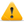A rectangle has a width of x and a length of 4x

Write down a formula for the perimeter (P) in terms of x. Ensure the formula is in its simplest terms.The correct answer is: P = 10xThis is a sample question. You can start the practice exam by clicking the 'Start Practice Exam' link on the exam page.

Back to practice exam

Free practice exams are provided free of charge by Accelerated Ideas. Please respect our exams and do not reproduce our material or abuse the testing system. Thank you. Maths practice tests for SAT Maths and GCSE Maths
Home